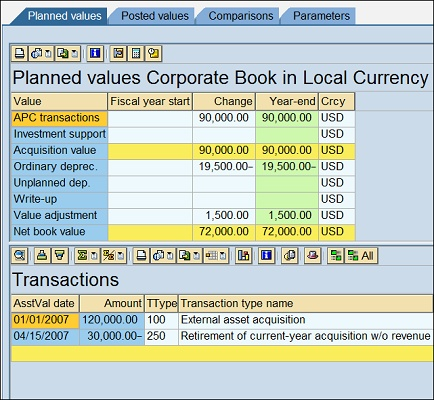# Details on SAP’s New Depreciation Engine (Part 2): Explanation of Time Intervals

```Blog Series:
Details on SAP's New Depreciation Engine (Part 1): Introduction
Details on SAP's New Depreciation Engine (Part 2): Explanation of Time Intervals
Details on SAP's New Depreciation Engine (Part 3): Time-Dependent Depreciation Parameters
Details on SAP's New Depreciation Engine (Part 4): Changes to the Asset Explorer```

In my previous blog I introduced the New Depreciation Calculation (also referred to as NewDCP) that is delivered in ERP 6.0 as part of the EA-FIN Enterprise Extension. This blog will go into more detail and provide some examples of the specific change in how asset planned amounts are now calculated.

### Overview of “Old” Depreciation Calculation

First, let’s review how the system calculated depreciation in prior releases.

In prior R/3 releases, SAP calculated depreciation on a transaction line item level. Every time a transaction is posted to the asset — either an acquisition, retirement or transfer — SAP calculated the depreciation amounts associated with that line item. For example, an asset could receive several dozen transactions over the course of its lifetime and each one would be uniquely valued from a depreciation perspective.

If you look at the image below of table ANEP from a R/3 4.7 system, you’ll see an asset with multiple acquisitions and retirements. In the highlighted column you can see how the system has calculated a corresponding depreciation amount for each unique posting. You could also view this information at the Asset Explorer as well as an Asset Transaction report such as RABEWG.### Overview of “New” Depreciation Calculation

As of ERP 6.0 this transaction based approach to depreciation calculation is no longer used. Instead the asset’s depreciation value is determined by evaluating period intervals. A period interval is created for each asset, depreciation type (i.e. Ordinary Depreciation), depreciation area and fiscal year. New intervals are created whenever something happens that changes the asset’s value. Most of the time this is triggered by new transaction postings but it can also be triggered based on other changes. Two examples are if a time dependent depreciation parameter is changed (ex. useful life) or a multi-level method change occurs within the fiscal year. If there are no changes to the asset’s value then only a single interval will exist… but if a posting occurs, then a new interval is created and the first one adjusted (i.e., shortened).

### Example

As with most things in fixed assets, this is best explained with an example and some simple calculations. Here are the following assumptions for my example asset:

Fiscal Year = K4
Ordinary, straight line depreciation
Depreciation Base Value = 01 APC
Depreciation Method = Percentage from UL
Useful Life = 5 years
Period control = pro rata for all transactions

First Posting: The asset is acquired on January 2007 with an initial posting of \$120,000 USD. This results in a planned annual depreciation amount of \$24,000 based on the asset’s 5 year UL. Here’s how it looks at the Asset Explorer.To see the calculation in more detail navigate to the depreciation calculation from the Asset Explorer. This depreciation trace is another area that has been completely changed in 6.0 to account for the new calculation technique.

As you can see in the image below, the total planned depreciation for area 01 is shown as \$24,000. The bottom half of the image shows the components of the calculation and is the best way to confirm that the calculation is correct… the base value of \$120,000, the percentage rate of 20%, etc. Pay particular attention to the Period Factor. At this time the value is 1 because the calculation parameters are in effect for the entire fiscal year (12 out of 12 periods)… but that will change with our next posting.Second Posting: On April 18th, a partial retirement of \$30,000 USD is recorded. The image below from the Asset Explorer shows the second transaction and that the new planned annual depreciation amount has been adjusted down to \$19,500.Looking again at the detailed calculation we can see how this annual amount of \$19,500 was determined.The retirement posting has the following effects on the depreciation calculation. First, it reduces the base value from \$120,000 to \$90,000. In past releases with the old depreciation calculation, the 2nd posting would be valuated using the amount of the transaction (minus \$30,000 in this case) which would result in a positive adjustment to the initially calculated depreciation amount of \$24,000. The new depreciation calculation instead focuses on the base value of the asset that is in effect for that period interval. Based on the configuration of the asset, the base value is set to the total APC value so \$90,000 is used to calculate the depreciation amount.

The second thing to note is the changes to the Period Factor. Since the date of the retirement transaction is in April and the period control is pro rata, the effect of the change is in period 4. This shortens the first interval so that it is now only in effect for periods 1-3. This second posting has created a new depreciation period interval that lasts for the remaining periods 4-12. The period factor (which is essentially the period duration) for the first interval is now .25 (period length of 3 / total of 12 periods) and the 2nd interval is for the remaining .75 (9 / 12).

### What’s next?

In the next blog I’ll go into more detail about the time-dependent depreciation parameters that are now maintainable on the fixed asset record.

```Blog Series:
Details on SAP's New Depreciation Engine (Part 1): Introduction
Details on SAP's New Depreciation Engine (Part 2): Explanation of Time Intervals
Details on SAP's New Depreciation Engine (Part 3): Time-Dependent Depreciation Parameters
Details on SAP's New Depreciation Engine (Part 4): Changes to the Asset Explorer```